##### Trigonometry Workbook For DummiesIn the olden days, knowing how to divide large numbers was important. Basic long division is still good to know, so the following examples will show you how to divide a one-digit divisor into another number, and then how to find a remainder.

Recall that the divisor in a division problem is the number that you’re dividing by. When you’re doing long division, the size of the divisor is your main concern: Small divisors are easy to work with, and large ones are a royal pain. So here you’ll work with a nice, small, one-digit divisor. Suppose you want to find 860 5. Start off by writing the problem like this: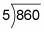Unlike the other Big Four operations, long division moves from left to right. In this case, you start with the number in the hundreds column (8). To begin, ask how many times 5 goes into 8 — that is, what’s 8 5? The answer is 1 (with a little bit left over), so write 1 directly above the 8. Now multiply 1 5 to get 5, place the answer directly below the 8, and draw a line beneath it: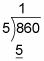Subtract 8 – 5 to get 3. (Note: After you subtract, the result should always be smaller than the divisor. If not, you need to write a higher number above the division symbol.) Then bring down the 6 to make the new number 36: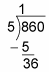These steps are one complete cycle, and to complete the problem you just need to repeat them. Now ask how many times 5 goes into 36 — that is, what’s 36 5? The answer is 7 (with a little left over). Write 7 just above the 6, and then multiply 7 5 to get 35; write the answer under 36: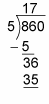Now subtract to get 36 – 35 = 1; bring down the 0 next to the 1 to make the new number 10: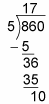Another cycle is complete, so begin the next cycle by asking how many times 5 goes into 10 — that is, 10 5. The answer this time is 2. Write down the 2 in the answer above the 0. Multiply to get 2 5 = 10, and write this answer below the 10: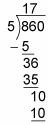Now subtract 10 – 10 = 0. Because you have no more numbers to bring down, you’re finished, and here’s the answer (that is, the quotient):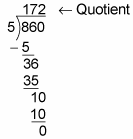So 860 5 = 172.

This problem divides evenly, but many don’t. The following instructions tell you what to do when you run out of numbers to bring down.

Division is different from addition, subtraction, and multiplication in that having a remainder is possible. A remainder is simply a portion left over from the division.

The letter r indicates that the number that follows is the remainder.

For example, suppose you want to divide seven candy bars between two people without breaking any candy bars into pieces (too messy). So each person receives three candy bars, and one candy bar is left over. This problem shows you the following:

7 2 = 3 with a remainder of 1, or 3 r 1

In long division, the remainder is the number that’s left when you no longer have numbers to bring down. The following equation shows that 47 3 = 15 r 2: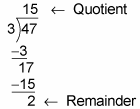Note that when you’re doing division with a small dividend and a larger divisor, you always get a quotient of 0 and a remainder of the number you started with:

1 2 = 0 r 1

14 23 = 0 r 14

2,000 2,001 = 0 r 2,000